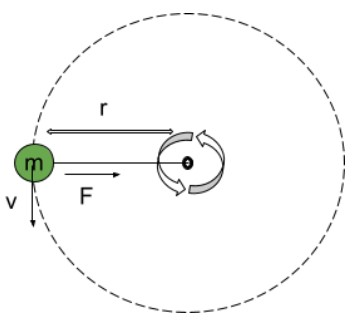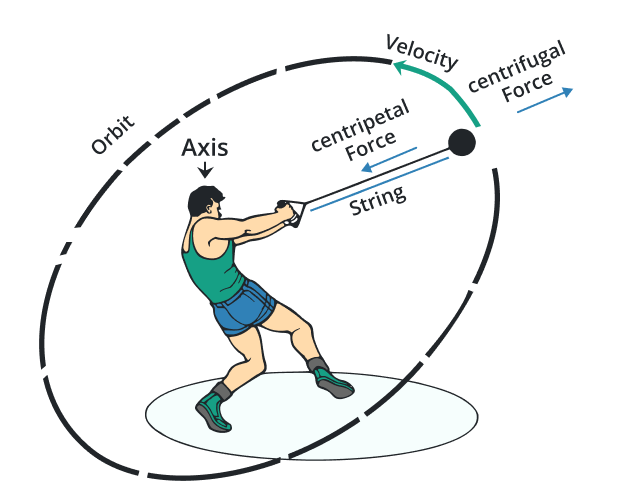# Centripetal Force Calculator

To calculate centripetal force, put the values of the mass, velocity, and radius in the centripetal force calculator.

ms-1

Formula

Give Us Feedback

## Centripetal Force Calculator

Centripetal force calculator is an online tool that calculates the centripetal force by using mass, velocity, and radius.

## What is centripetal force?The definition of centripetal force:

“It is a force, directed towards the center that keeps a body in a circular path.”

The unit of centripetal force is Newton or kgms-2.## Centripetal force formula

The equation for centripetal force is,

Fc =mv2/r

In this equation:

• F represents the centripetal force,
• m is mass,
• V is velocity, and

## How to calculate centripetal force?

Example:

A kid is rotating a ball of a mass of 10 kg attached to a string of 2 meters in a circular motion with a velocity of 4ms-1. Calculate the centripetal force acting on the ball.

Solution:

Step 1: Identify the values.

V = 4ms-1

r = 2 m

m = 10 kg

Step 2: Put the values in the formula of centripetal force:

Fc = mv2/r

Fc = (10)(42)/2

Fc = 160/2

Fc = 80 N

### Math Tools